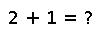# get

``````..  get(f::Function, collection, key)

Return the value stored for the given key, or if no mapping for the key is present, return ``f()``.  Use :func:`get!` to also store the default value in the dictionary.

This is intended to be called using ``do`` block syntax::

get(dict, key) do
# default value calculated here
time()
end``````

## Examples

``````julia> dict = ["A"=>1, "B"=>2];

julia> get(dict, "A", 42)
1

julia> get(dict, "C", 42)
42

julia> get(() -> "Hello", dict, "D")
"Hello"``````

#### `do` block syntax

``````julia> function f(key)
get(dict,key) do        # dict from above
reduce(+,[1:10])    # = 1 + 2 + 3 ..
end
end;
julia> f("B")
2
julia> f("foo")
55``````
``````julia> dict = Dict(["one"=> 1, "two"=> 2, "three"=> 3])
get(dict,"one",5)
1``````
``````julia> dict = Dict(["one"=> 1, "two"=> 2, "three"=> 3])
get(dict,"four",5)
5``````

Checking you are not a robot: